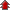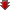# NCERT Solutions for Class 12th Chemistry

• NCERT Chapter### Exercise 1

•  Q1 Why are solids rigid? Q2 Why do solids have a definite volume? Q3 Classify the following as amorphous or crystalline solids: Polyurethane, naphthalene, benzoic acid, teflon, potassium nitrate, cellophane, polyvinyl chloride, fibre glass, copper. Q4 Why is glass considered a super cooled liquid? Q5 Refractive index of a solid is observed to have the same value along all directions. Comment on the nature of this solid. Would it show cleavage property? Q6 Classify the following solids in different categories based on the nature of intermolecular forces operating in them: Potassium sulphate, tin, benzene, urea, ammonia, water, zinc sulphide, graphite, rubidium, argon, silicon carbide. Q7 Solid A is a very hard electrical insulator in solid as well as in molten state and melts at extremely high temperature. What type of solid is it? Q8 Ionic solids conduct electricity in molten state but not in solid state. Explain. Q9 What type of solids are electrical conductors, malleable and ductile? Q10 Give the significance of a lattice point. Q11 Name the parameters that characterize a unit cell. Q12 Distinguish between (i)Hexagonal and monoclinic unit cells (ii) Face-centred and end-centred unit cells. Q13 Explain how much portion of an atom located at (i) corner and (ii) body-centre of a cubic unit cell is part of its neighbouring unit cell.">Explain how much portion of an atom located at (i) corner and (ii) body-centre of a cubic unit cell is part of its neighbouring unit cell. Q14 What is the two dimensional coordination number of a molecule in square close packed layer? Q15 A compound forms hexagonal close-packed structure. What is the total number of voids in 0.5 mol of it? How many of these are tetrahedral voids?">A compound forms hexagonal close-packed structure. What is the total number of voids in 0.5 mol of it? How many of these are tetrahedral voids? Q16 A compound is formed by two elements M and N. The element N forms ccp and atoms of M occupy 1/3rd of tetrahedral voids. What is the formula of the compound?">A compound is formed by two elements M and N. The element N forms ccp and atoms of M occupy 1/3rd of tetrahedral voids. What is the formula of the compound? Q17 Which of the following lattices has the highest packing efficiency (i) simple cubic (ii) body-centred cubic and (iii) hexagonal close-packed lattice?">Which of the following lattices has the highest packing efficiency (i) simple cubic (ii) body-centred cubic and (iii) hexagonal close-packed lattice? Q18 An element with molar mass 2.7 x 10-2kg mol-1 forms a cubic unit cell with edge length 405 pm. If its density is 2.7 x 103 kg m-3, what is the nature of the cubic unit cell?">An element with molar mass 2.7 x 10-2kg mol-1 forms a cubic unit cell with edge length 405 pm. If its density is 2.7 x 103 kg m-3, what is the nature of the cubic unit cell? Q19 What type of defect can arise when a solid is heated? Which physical property is affected by it and in what way? Q20 What type of stoichiometric defect is shown by: (i) ZnS (ii) AgBr Q21 Explain how vacancies are introduced in an ionic solid when a cation of higher valence is added as an impurity in it.">Explain how vacancies are introduced in an ionic solid when a cation of higher valence is added as an impurity in it. Q22 Ionic solids, which have anionic vacancies due to metal excess defect, develop colour. Explain with the help of a suitable example.">Ionic solids, which have anionic vacancies due to metal excess defect, develop colour. Explain with the help of a suitable example. Q23 A group 14 element is to be converted into n-type semiconductor by doping it with a suitable impurity. To which group should this impurity belong?">A group 14 element is to be converted into n-type semiconductor by doping it with a suitable impurity. To which group should this impurity belong? Q24 What type of substances would make better permanent magnets, ferromagnetic or ferrimagnetic. Justify your answer.">What type of substances would make better permanent magnets, ferromagnetic or ferrimagnetic. Justify your answer.